Anúncio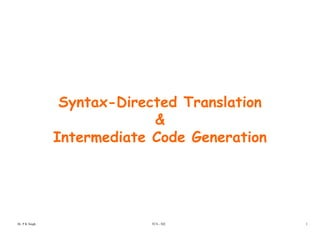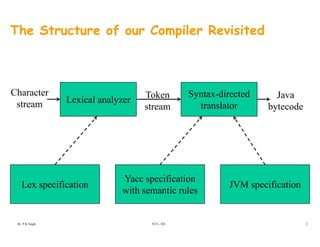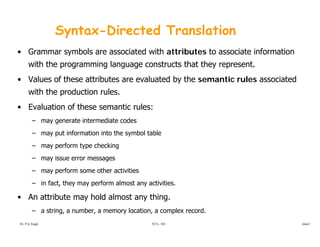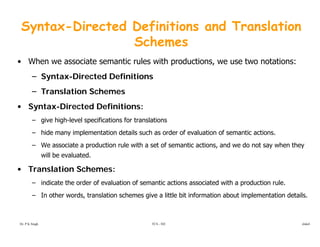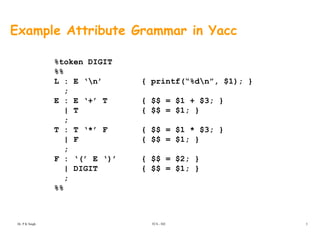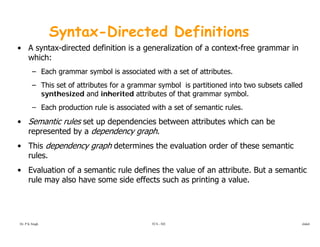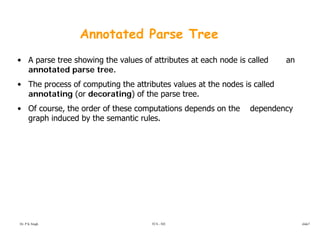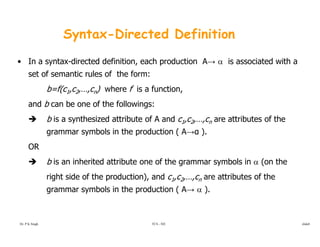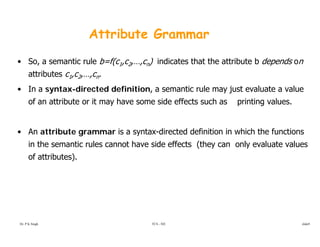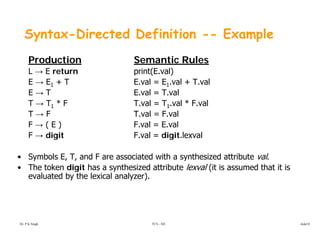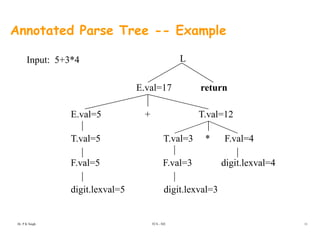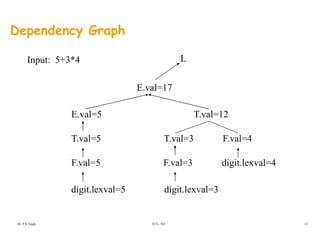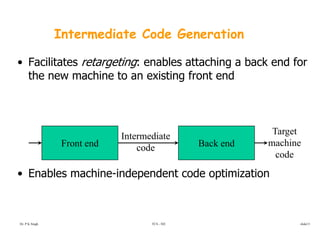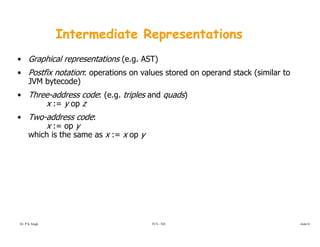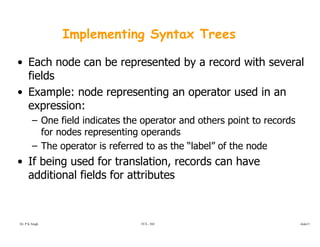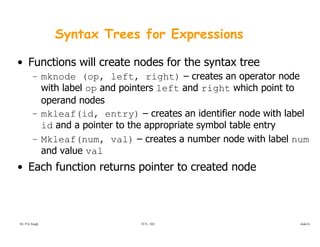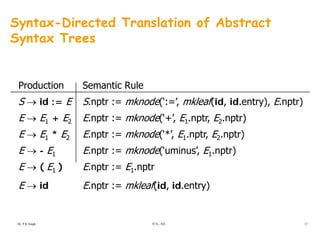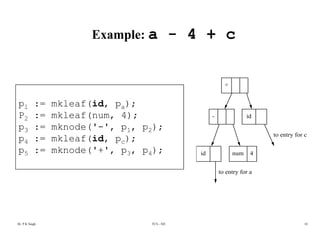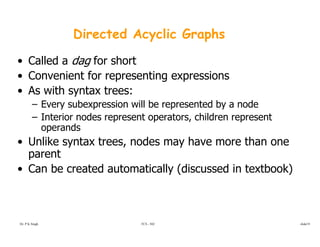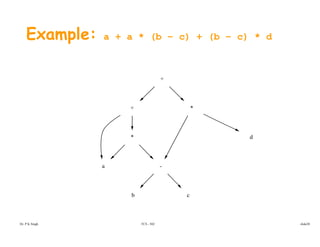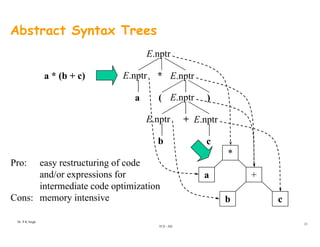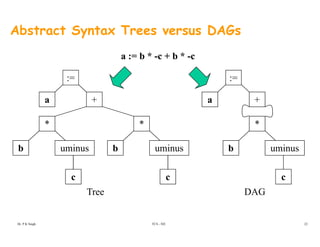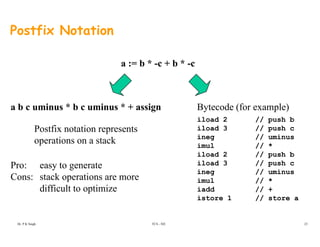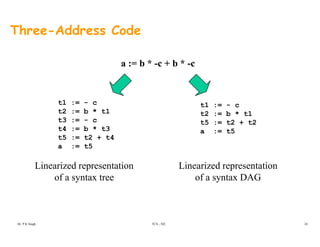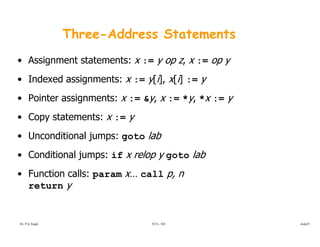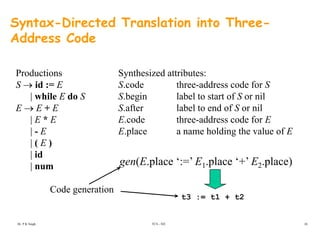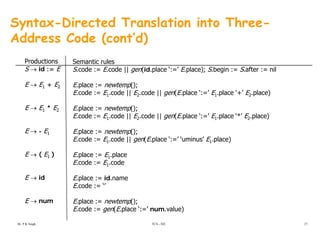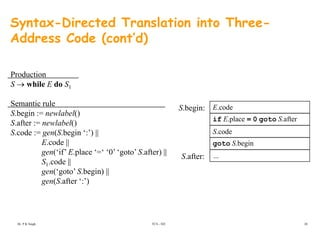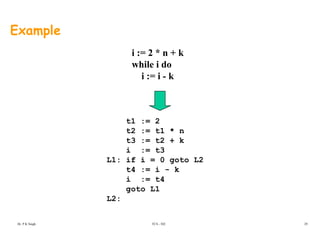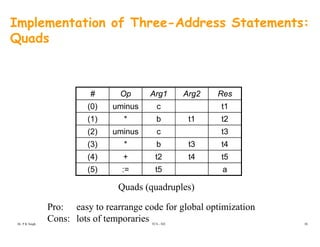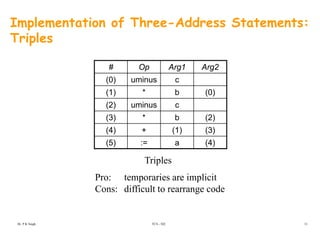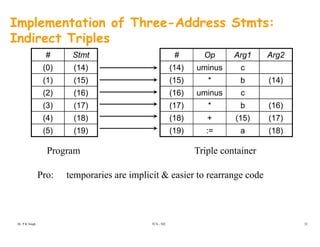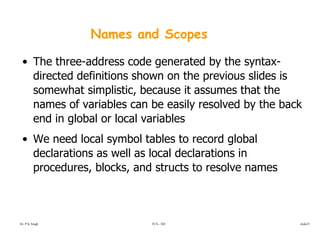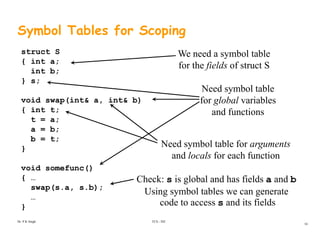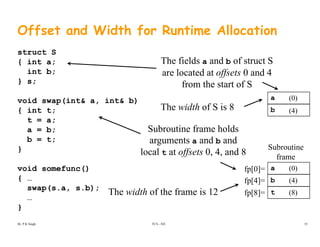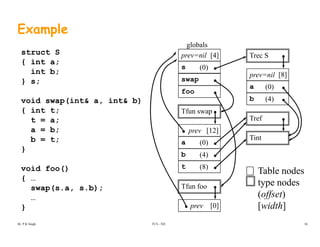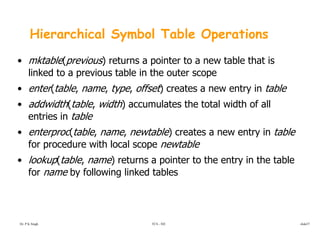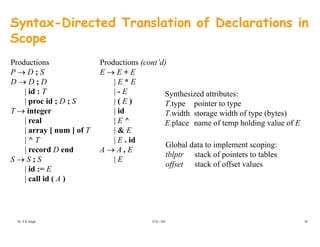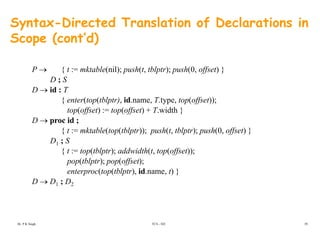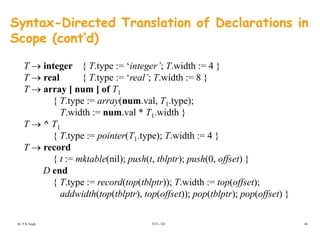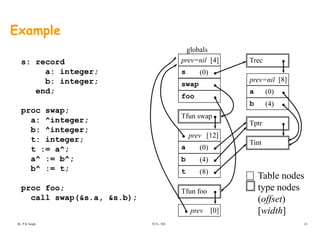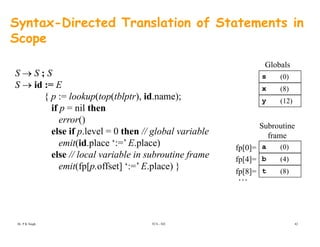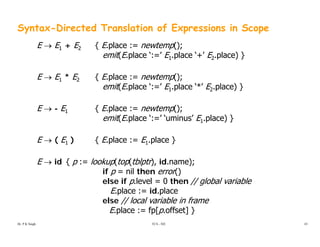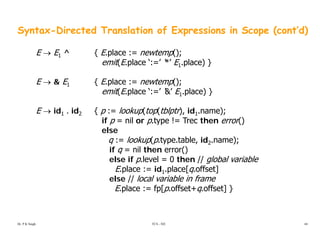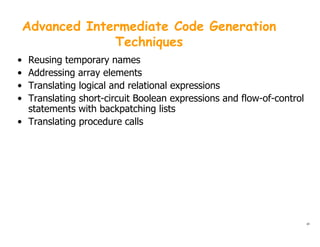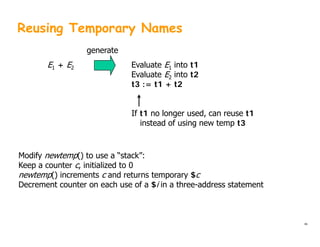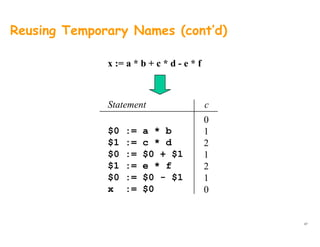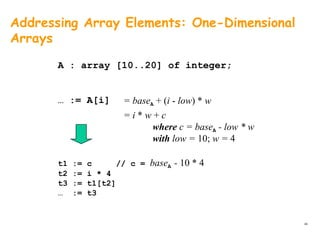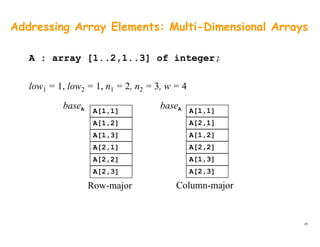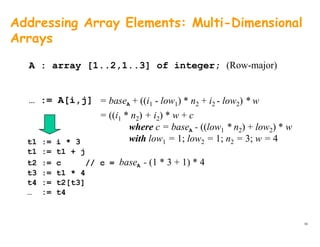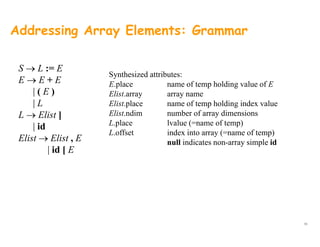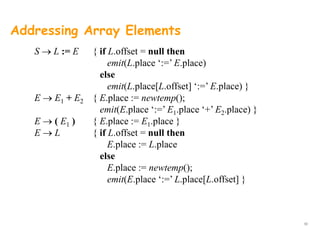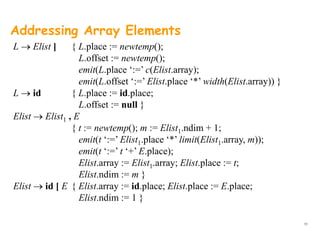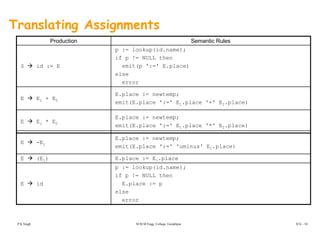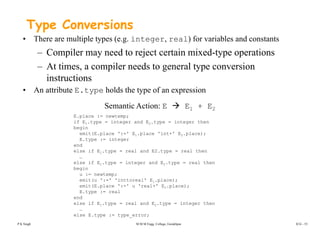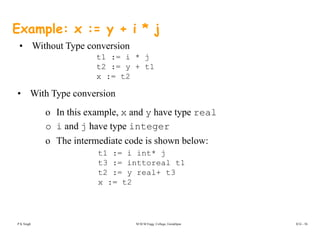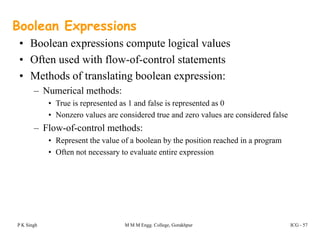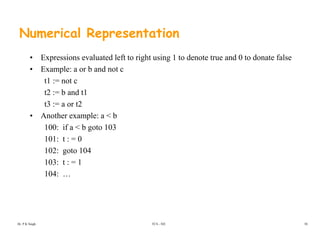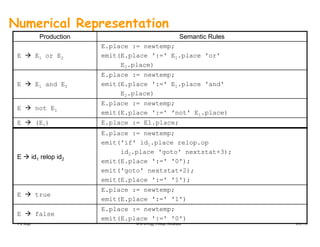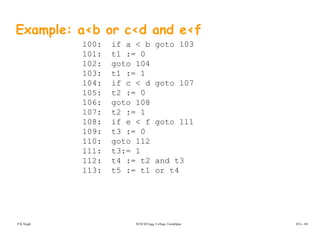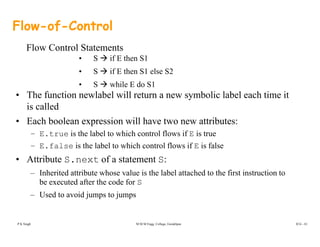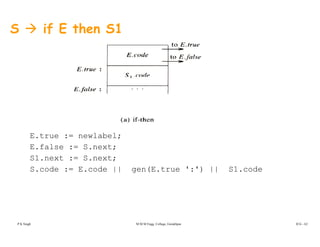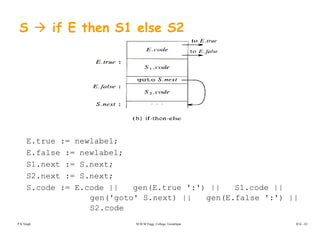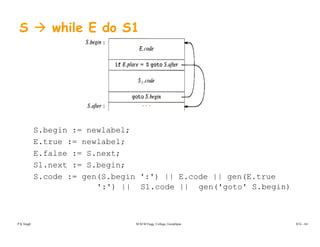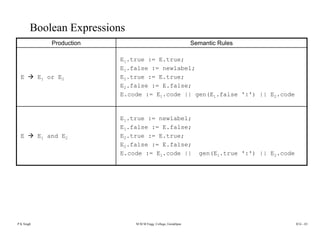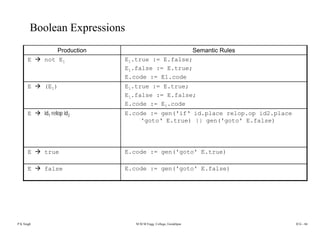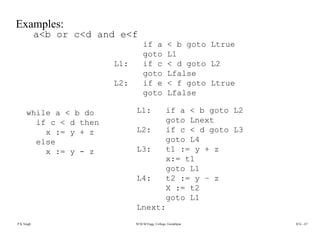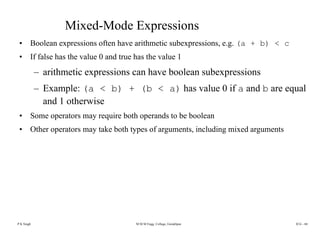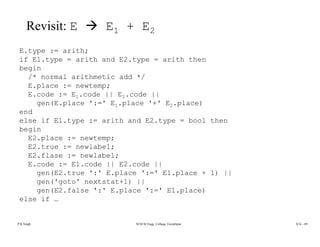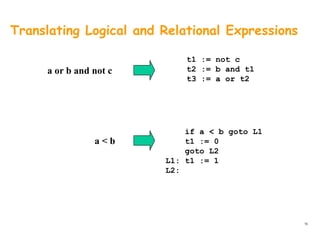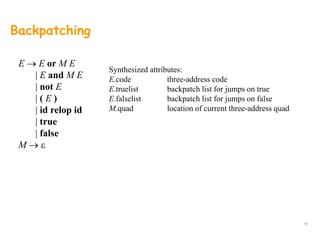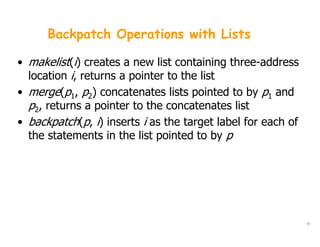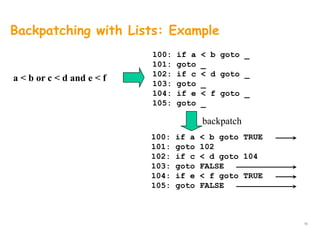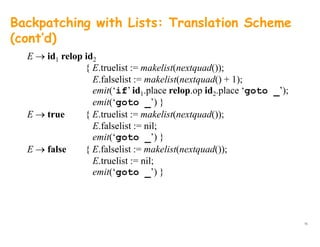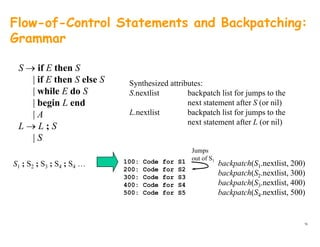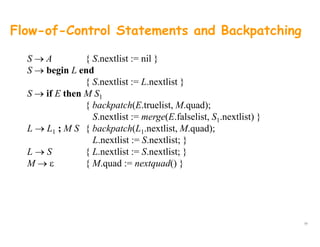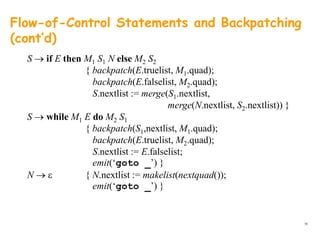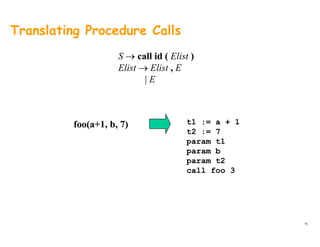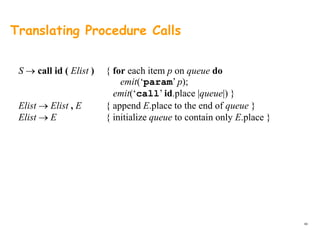1 de 80
Anúncio

### Syntaxdirected

1. Syntax-Directed Translationy & Intermediate Code GenerationIntermediate Code Generation TCS - 502Dr. P K Singh 1
2. The Structure of our Compiler Revisited Lexical analyzer Syntax-directed translator Character stream Token stream Java bytecodetranslatorstream bytecode Yacc specification with semantic rules JVM specificationLex specification TCS - 502Dr. P K Singh 2
3. Syntax-Directed Translation • Grammar symbols are associated with attributes to associate information with the programming language constructs that they represent. V l f th tt ib t l t d b th ti l i t d• Values of these attributes are evaluated by the semantic rules associated with the production rules. • Evaluation of these semantic rules:• Evaluation of these semantic rules: – may generate intermediate codes – may put information into the symbol table – may perform type checking – may issue error messages may perform some other activities– may perform some other activities – in fact, they may perform almost any activities. • An attribute may hold almost any thing. TCS - 502 y y g – a string, a number, a memory location, a complex record. Dr. P K Singh slide3
4. Syntax-Directed Definitions and Translation SchemesSchemes • When we associate semantic rules with productions, we use two notations: – Syntax-Directed Definitions– Syntax-Directed Definitions – Translation Schemes • Syntax-Directed Definitions:• Syntax Directed Definitions: – give high-level specifications for translations – hide many implementation details such as order of evaluation of semantic actions. – We associate a production rule with a set of semantic actions, and we do not say when they will be evaluated. • Translation Schemes:• Translation Schemes: – indicate the order of evaluation of semantic actions associated with a production rule. – In other words, translation schemes give a little bit information about implementation details. TCS - 502Dr. P K Singh slide4
5. Example Attribute Grammar in Yacc %token DIGIT %% L : E ‘n’ { printf(“%dn”, $1); } ; E : E ‘+’ T { $$= 1 + 3; } | {$$$1 }| T { $$= 1; } ; T : T ‘*’ F {$$ = $1 *$3; } | F { $$= 1; }| F {$$ = $1; } ; F : ‘(’ E ‘)’ { $$= 2; } | DIGIT {$$ =$1; }| DIGIT {  $1; } ; %% TCS - 502Dr. P K Singh 5 6. Syntax-Directed Definitions A t di t d d fi iti i li ti f t t f i• A syntax-directed definition is a generalization of a context-free grammar in which: – Each grammar symbol is associated with a set of attributes. – This set of attributes for a grammar symbol is partitioned into two subsets called synthesized and inherited attributes of that grammar symbol. – Each production rule is associated with a set of semantic rulesEach production rule is associated with a set of semantic rules. • Semantic rules set up dependencies between attributes which can be represented by a dependency graph. • This dependency graph determines the evaluation order of these semantic rules. • Evaluation of a semantic rule defines the value of an attribute But a semantic• Evaluation of a semantic rule defines the value of an attribute. But a semantic rule may also have some side effects such as printing a value. TCS - 502Dr. P K Singh slide6 7. Annotated Parse Tree • A parse tree showing the values of attributes at each node is called an annotated parse tree. • The process of computing the attributes values at the nodes is called annotating (or decorating) of the parse tree. • Of course the order of these computations depends on the dependency• Of course, the order of these computations depends on the dependency graph induced by the semantic rules. TCS - 502Dr. P K Singh slide7 8. Syntax-Directed Definition • In a syntax-directed definition, each production A→ α is associated with a set of semantic rules of the form:set of semantic rules of the form: b=f(c1,c2,…,cn) where f is a function, and b can be one of the followings:g b is a synthesized attribute of A and c1,c2,…,cn are attributes of the grammar symbols in the production ( A→α ). OR b is an inherited attribute one of the grammar symbols in α (on the right side of the production), and c1,c2,…,cn are attributes of the grammar symbols in the production ( A→ α ). TCS - 502Dr. P K Singh slide8 9. Attribute Grammar • So, a semantic rule b=f(c1,c2,…,cn) indicates that the attribute b depends on attributes c1,c2,…,cn.attributes c1,c2,…,cn. • In a syntax-directed definition, a semantic rule may just evaluate a value of an attribute or it may have some side effects such as printing values. • An attribute grammar is a syntax-directed definition in which the functions in the semantic rules cannot have side effects (they can only evaluate values of attributes). TCS - 502Dr. P K Singh slide9 10. Syntax-Directed Definition -- Example Production Semantic Rules L → E return print(E.val) E → E1 + T E.val = E1.val + T.val E → T E.val = T.val T → T1 * F T.val = T1.val * F.val1 1 T → F T.val = F.val F → ( E ) F.val = E.val F → digit F.val = digit.lexvalg g • Symbols E, T, and F are associated with a synthesized attribute val. • The token digit has a synthesized attribute lexval (it is assumed that it ise to e d g t as a sy t es ed att bute e a ( t s assu ed t at t s evaluated by the lexical analyzer). TCS - 502Dr. P K Singh slide10 11. Annotated Parse Tree -- Example Input: 5+3*4 L E.val=17 return E.val=5 + T.val=12 T val=5 T val=3 * F val=4T.val=5 T.val=3 F.val=4 F.val=5 F.val=3 digit.lexval=4 digit.lexval=5 digit.lexval=3 TCS - 502Dr. P K Singh 11 12. Dependency Graph Input: 5+3*4 L E.val=17 E.val=5 T.val=12 T val=5 T val=3 F val=4T.val=5 T.val=3 F.val=4 F.val=5 F.val=3 digit.lexval=4 digit.lexval=5 digit.lexval=3 TCS - 502Dr. P K Singh 12 13. Intermediate Code Generation • Facilitates retargeting: enables attaching a back end for the new machine to an existing front endthe new machine to an existing front end Intermediate Target E bl hi i d d t d ti i ti Front end Back end Intermediate code machine code • Enables machine-independent code optimization TCS - 502Dr. P K Singh slide13 14. Intermediate Representations • Graphical representations (e.g. AST) • Postfix notation: operations on values stored on operand stack (similar top p ( JVM bytecode) • Three-address code: (e.g. triples and quads) x := y op zy p • Two-address code: x := op y which is the same as x := x op y TCS - 502Dr. P K Singh slide14 15. Implementing Syntax Trees • Each node can be represented by a record with several fieldsfields • Example: node representing an operator used in an expression:expression: – One field indicates the operator and others point to records for nodes representing operands – The operator is referred to as the “label” of the node • If being used for translation, records can have f fadditional fields for attributes Dr. P K Singh TCS - 502 slide15 16. Syntax Trees for Expressions • Functions will create nodes for the syntax tree – mknode (op left right) – creates an operator node– mknode (op, left, right) creates an operator node with label op and pointers left and right which point to operand nodes – mkleaf(id, entry) – creates an identifier node with label id and a pointer to the appropriate symbol table entry Mkleaf(num val) – creates a number node with label num– Mkleaf(num, val) – creates a number node with label num and value val • Each function returns pointer to created nodeEach function returns pointer to created node Dr. P K Singh TCS - 502 slide16 17. Syntax-Directed Translation of Abstract Syntax Treesy Production S → id := E Semantic Rule S.nptr := mknode(‘:=’, mkleaf(id, id.entry), E.nptr) E → E1 + E2 E → E1 * E2 E.nptr := mknode(‘+’, E1.nptr, E2.nptr) E.nptr := mknode(‘*’, E1.nptr, E2.nptr)1 2 E → - E1 E → ( E1 ) p ( , 1 p , 2 p ) E.nptr := mknode(‘uminus’, E1.nptr) E.nptr := E1.nptr→ ( 1 ) E → id pt 1 pt E.nptr := mkleaf(id, id.entry) TCS - 502Dr. P K Singh 17 18. Example: a - 4 + c p1 := mkleaf(id, pa); + p1 pa P2 := mkleaf(num, 4); p3 := mknode('-', p1, p2); p := mkleaf(id p ); - id to entry for c p4 := mkleaf(id, pc); p5 := mknode('+', p3, p4); id num 4 to entry for ato entry for a Dr. P K Singh TCS - 502 18 19. Directed Acyclic Graphs • Called a dag for short • Convenient for representing expressions• Convenient for representing expressions • As with syntax trees: – Every subexpression will be represented by a nodey p p y – Interior nodes represent operators, children represent operands U lik t t d h th• Unlike syntax trees, nodes may have more than one parent • Can be created automatically (discussed in textbook)• Can be created automatically (discussed in textbook) Dr. P K Singh TCS - 502 slide19 20. Example: a + a * (b – c) + (b – c) * d ++ + *+ * * d a * - d b c Dr. P K Singh TCS - 502 slide20 21. Abstract Syntax Trees E.nptr *E nptr E nptra * (b + c) E.nptr E.nptra E.nptr ( ) a (b + c) b +E.nptr E.nptr b * c Pro: easy restructuring of code a + b c and/or expressions for intermediate code optimization Cons: memory intensive b cy TCS - 502 Dr. P K Singh 21 22. Abstract Syntax Trees versus DAGs : : a := b * -c + b * -c := a + := a + * * * uminusb uminusb uminusb c c c Tree DAG TCS - 502Dr. P K Singh 22 23. Postfix Notation a := b * -c + b * -c a b c uminus * b c uminus * + assign Bytecode (for example)a b c uminus b c uminus + assign iload 2 // push b iload 3 // push c ineg // uminus Bytecode (for example) Postfix notation represents ti t k ineg // uminus imul // * iload 2 // push b iload 3 // push c i // i operations on a stack Pro: easy to generate ineg // uminus imul // * iadd // + istore 1 // store a y g Cons: stack operations are more difficult to optimize TCS - 502Dr. P K Singh 23 24. Three-Address Code a := b * -c + b * -c t1 := - c t1 := - c t2 := b * t1 t3 := - c t4 := b * t3 t5 := t2 + t4 t2 := b * t1 t5 := t2 + t2 a := t5 t5 : t2 + t4 a := t5 Linearized representation Linearized representationp of a syntax tree p of a syntax DAG TCS - 502Dr. P K Singh 24 25. Three-Address Statements • Assignment statements: x := y op z, x := op y I d d i t [i] [i]• Indexed assignments: x := y[i], x[i] := y • Pointer assignments: x := &y, x := *y, *x := y • Copy statements: x := y • Unconditional jumps: goto labUnconditional jumps: goto lab • Conditional jumps: if x relop y goto lab F ti ll• Function calls: param x… call p, n return y TCS - 502Dr. P K Singh slide25 26. Syntax-Directed Translation into Three- Address Code Synthesized attributes:Productions y S.code three-address code for S S.begin label to start of S or nil S after label to end of S or nil S → id := E | while E do S E → E + E S.after label to end of S or nil E.code three-address code for E E.place a name holding the value of E E → E + E | E * E | - E | ( E ) | id | num gen(E.place ‘:=’ E1.place ‘+’ E2.place)| t3 := t1 + t2 Code generation TCS - 502Dr. P K Singh 26 27. Syntax-Directed Translation into Three- Address Code (cont’d) Productions S → id := E Semantic rules S.code := E.code || gen(id.place ‘:=’ E.place); S.begin := S.after := nil E → E1 + E2 E → E * E E.place := newtemp(); E.code := E1.code || E2.code || gen(E.place ‘:=’ E1.place ‘+’ E2.place) E place := newtemp();E → E1 * E2 E → - E1 E.place := newtemp(); E.code := E1.code || E2.code || gen(E.place ‘:=’ E1.place ‘*’ E2.place) E.place := newtemp(); E code := E code || gen(E place ‘:=’ ‘uminus’ E place) E → ( E1 ) E.code := E1.code || gen(E.place := uminus E1.place) E.place := E1.place E.code := E1.code E → id E → num E.place := id.name E.code := ‘’ E place := newtemp();E → num E.place := newtemp(); E.code := gen(E.place ‘:=’ num.value) TCS - 502Dr. P K Singh 27 28. Syntax-Directed Translation into Three- Address Code (cont’d) ProductionProduction S → while E do S1 Semantic rule E codeS begin: S.begin := newlabel() S.after := newlabel() S.code := gen(S.begin ‘:’) || if E.place = 0 goto S.after S.code E.codeS.begin: E.code || gen(‘if’ E.place ‘=‘ ‘0’ ‘goto’ S.after) || S1.code || (‘ t ’ S b i ) || … goto S.begin S.after: gen(‘goto’ S.begin) || gen(S.after ‘:’) TCS - 502Dr. P K Singh 28 29. Example i := 2 * n + k while i do i := i - ki := i - k t1 := 2 t2 := t1 * n t3 t2 + kt3 := t2 + k i := t3 L1: if i = 0 goto L2 t4 := i - kt4 := i k i := t4 goto L1 L2: TCS - 502Dr. P K Singh 29 30. Implementation of Three-Address Statements: Quads # Op Arg1 Arg2 Res (0) uminus c t1(0) uminus c t1 (1) * b t1 t2 (2) uminus c t3 (3) * b t3 t4 (4) + t2 t4 t5 (5) := t5 a(5) := t5 a Quads (quadruples) Pro: easy to rearrange code for global optimization Cons: lots of temporaries TCS - 502Dr. P K Singh 30 31. Implementation of Three-Address Statements: Triplesp # Op Arg1 Arg2 (0) i(0) uminus c (1) * b (0) (2) uminus c( ) (3) * b (2) (4) + (1) (3) (5) := a (4) Triples Pro: temporaries are implicit Cons: difficult to rearrange code TCS - 502Dr. P K Singh 31 32. Implementation of Three-Address Stmts: Indirect Triplesp # Op Arg1 Arg2 (14) uminus c # Stmt (0) (14) (15) * b (14) (16) uminus c (17) * b (16) (1) (15) (2) (16) (3) (17) (17) b (16) (18) + (15) (17) (19) := a (18) (3) (17) (4) (18) (5) (19) Triple containerProgram Pro: temporaries are implicit & easier to rearrange code TCS - 502Dr. P K Singh 32 33. Names and Scopes • The three-address code generated by the syntax- directed definitions shown on the previous slides isdirected definitions shown on the previous slides is somewhat simplistic, because it assumes that the names of variables can be easily resolved by the backnames of variables can be easily resolved by the back end in global or local variables • We need local symbol tables to record global• We need local symbol tables to record global declarations as well as local declarations in procedures, blocks, and structs to resolve namesprocedures, blocks, and structs to resolve names TCS - 502Dr. P K Singh slide33 34. Symbol Tables for Scoping struct S { int a; int b; We need a symbol table for the fields of struct S } s; void swap(int& a, int& b) { i t t Need symbol table for global variables { int t; t = a; a = b; b = t; and functions b = t; } void somefunc() Need symbol table for arguments and locals for each function void somefunc() { … swap(s.a, s.b); … Check: s is global and has fields a and b Using symbol tables we can generate code to access s and its fields} code to access s and its fields TCS - 502Dr. P K Singh 34 35. Offset and Width for Runtime Allocation struct S { int a; int b; The fields a and b of struct S are located at offsets 0 and 4 } s; void swap(int& a, int& b) { i t t a b (0) ff from the start of S The width of S is 8{ int t; t = a; a = b; b = t; Subroutine frame holds arguments and b and b (4)The width of S is 8 b = t; } void somefunc() arguments a and b and local t at offsets 0, 4, and 8 a (0) Subroutine frame fp=void somefunc() { … swap(s.a, s.b); … a b t (0) (4) (8) fp fp= fp=The width of the frame is 12 } TCS - 502Dr. P K Singh 35 36. Example globals struct S { int a; int b; Trec S s prev=nil prev=nil globals (0)   } s; void swap(int& a, int& b) { i t t a b prev nil swap foo (0) (4)  { int t; t = a; a = b; b = t; Tint Tfun swap Tref prev  b = t; } void foo() Tint a b t (0) (4) (8) Table nodesvoid foo() { … swap(s.a, s.b); … Tfun foo ( ) Table nodes type nodes (offset) } prev [width] TCS - 502Dr. P K Singh 36 37. Hierarchical Symbol Table Operations • mktable(previous) returns a pointer to a new table that is linked to a previous table in the outer scopep p • enter(table, name, type, offset) creates a new entry in table • addwidth(table, width) accumulates the total width of all( , ) entries in table • enterproc(table, name, newtable) creates a new entry in table for procedure with local scope newtable • lookup(table, name) returns a pointer to the entry in the table fo name b follo ing linked tablesfor name by following linked tables TCS - 502Dr. P K Singh slide37 38. Syntax-Directed Translation of Declarations in Scopep Productions P → D ; S Productions (cont’d) E → E + E Synthesized attributes: T.type pointer to type D → D ; D | id : T | proc id ; D ; S | E * E | - E | ( E ) | T.width storage width of type (bytes) E.place name of temp holding value of E T → integer | real | array [ num ] of T | ^ T | id | E ^ | & E | E id| ^ T | record D end S → S ; S | id := E Global data to implement scoping: tblptr stack of pointers to tables offset stack of offset values | E . id A → A , E | E | id : E | call id ( A ) TCS - 502Dr. P K Singh 38 39. Syntax-Directed Translation of Declarations in Scope (cont’d)p P → { t := mktable(nil); push(t, tblptr); push(0, offset) } SD ; S D → id : T { enter(top(tblptr), id.name, T.type, top(offset)); ( ff ) ( ff ) T id h }top(offset) := top(offset) + T.width } D → proc id ; { t := mktable(top(tblptr)); push(t, tblptr); push(0, offset) } D SD1 ; S { t := top(tblptr); addwidth(t, top(offset)); pop(tblptr); pop(offset); t (t (tbl t ) id name t) }enterproc(top(tblptr), id.name, t) } D → D1 ; D2 TCS - 502Dr. P K Singh 39 40. Syntax-Directed Translation of Declarations in Scope (cont’d)p T → integer { T.type := ‘integer’; T.width := 4 } T → real { T type := ‘real’; T width := 8 }T → real { T.type := real ; T.width := 8 } T → array [ num ] of T1 { T.type := array(num.val, T1.type); T.width := num.val * T1.width } T → ^ T1 { T.type := pointer(T1.type); T.width := 4 }{ yp p ( 1 yp ); } T → record { t := mktable(nil); push(t, tblptr); push(0, offset) } D endD end { T.type := record(top(tblptr)); T.width := top(offset); addwidth(top(tblptr), top(offset)); pop(tblptr); pop(offset) } TCS - 502Dr. P K Singh 40 41. Example s: record a: integer; Trec s prev=nil globals (0)  b: integer; end; a b prev=nil swap foo (0) (4)  proc swap; a: ^integer; b: ^integer; t: integer; Tfun swap Tptr prev  t: integer; t := a^; a^ := b^; b^ := t; Tint a b t p (0) (4) (8) [ ] b : t; proc foo; call swap(&s.a, &s.b); t Tfun foo (8) Table nodes type nodes (offset)p prev (offset) [width] TCS - 502Dr. P K Singh 41 42. Syntax-Directed Translation of Statements in Scopep S → S ; S s (0) Globals ; S → id := E { p := lookup(top(tblptr), id.name); if p = nil then s x y (0) (8) (12) if p = nil then error() else if p.level = 0 then // global variable Subroutine frame emit(id.place ‘:=’ E.place) else // local variable in subroutine frame emit(fp[p.offset] ‘:=’ E.place) } a b t (0) (4) (8) fp= fp= f  ( p[p ] p ) } t (8)fp= … TCS - 502Dr. P K Singh 42 43. Syntax-Directed Translation of Expressions in Scope E → E + E { E place := newtemp();E → E1 + E2 { E.place := newtemp(); emit(E.place ‘:=’ E1.place ‘+’ E2.place) } E → E * E { E place := newtemp();E → E1 * E2 { E.place := newtemp(); emit(E.place ‘:=’ E1.place ‘*’ E2.place) } E → E { E place := newtemp();E → - E1 { E.place := newtemp(); emit(E.place ‘:=’ ‘uminus’ E1.place) } E → ( E ) { E place := E place }E → ( E1 ) { E.place := E1.place } E → id { p := lookup(top(tblptr), id.name); if p nil then error()if p = nil then error() else if p.level = 0 then // global variable E.place := id.place else // local variable in frameelse // local variable in frame E.place := fp[p.offset] } TCS - 502Dr. P K Singh 43 44. Syntax-Directed Translation of Expressions in Scope (cont’d) E → E1 ^ { E.place := newtemp(); emit(E.place ‘:=’ ‘*’ E1.place) } E → & E1 { E.place := newtemp(); emit(E.place ‘:=’ ‘&’ E1.place) } E id id { l k ( ( bl ) id )E → id1 . id2 { p := lookup(top(tblptr), id1.name); if p = nil or p.type != Trec then error() else q := lookup(p type table id name);q := lookup(p.type.table, id2.name); if q = nil then error() else if p.level = 0 then // global variable E place := id1 place[q offset]E.place := id1.place[q.offset] else // local variable in frame E.place := fp[p.offset+q.offset] } TCS - 502Dr. P K Singh 44 45. Advanced Intermediate Code Generation TechniquesTechniques • Reusing temporary names • Addressing array elementsg y • Translating logical and relational expressions • Translating short-circuit Boolean expressions and flow-of-control statements with backpatching listsstatements with backpatching lists • Translating procedure calls 45 46. Reusing Temporary Names Evaluate E1 into t1 Evaluate E2 into t2 E1 + E2 generate Evaluate E2 into t2 t3 := t1 + t2 If t1 no longer used, can reuse t1 instead of using new temp t3 Modify newtemp() to use a “stack”: Keep a counter c, initialized to 0 newtemp() increments c and returns temporary$c Decrement counter on each use of a $i in a three-address statement 46 47. Reusing Temporary Names (cont’d) x := a * b + c * d - e * f cStatement$0 := a * b $1 0 1$1 := c * d $0 :=$0 + $1$1 := e * f 2 1 2 $0 :=$0 - $1 x :=$0 1 0 47
48. Addressing Array Elements: One-Dimensional Arraysy A : array [10..20] of integer; = baseA + (i - low) * w… := A[i] = i * w + c where c = baseA - low * w with low = 10; w = 4with low = 10; w = 4 t1 := c // c = baseA - 10 * 4 t2 := i * 4 t3 := t1[t2] … := t3 48
49. Addressing Array Elements: Multi-Dimensional Arrays A : array [1..2,1..3] of integer; low1 = 1, low2 = 1, n1 = 2, n2 = 3, w = 4 baseA baseAA[1,1] A[1,2] A[1,3] A[1,1] A[2,1] A[1,2] baseA baseA A[2,1] A[2,2] A[2 3] A[2,2] A[1,3] A[2 3]A[2,3] Row-major A[2,3] Column-major 49
50. Addressing Array Elements: Multi-Dimensional Arraysy A : array [1..2,1..3] of integer; (Row-major) = baseA + ((i1 - low1) * n2 + i2 - low2) * w… := A[i,j] = ((i1 * n2) + i2) * w + c where c = baseA - ((low1 * n2) + low2) * w with low = 1; low = 1; n = 3; w = 41 3 with low1 = 1; low2 = 1; n2 = 3; w = 4t1 := i * 3 t1 := t1 + j t2 := c // c = baseA - (1 * 3 + 1) * 4 3 1 * 4t3 := t1 * 4 t4 := t2[t3] … := t4 50
51. Addressing Array Elements: Grammar S → L := E E E E Synthesized attributes: E → E + E | ( E ) | L Synthesized attributes: E.place name of temp holding value of E Elist.array array name Elist.place name of temp holding index value| L → Elist ] | id Elist → Elist E Elist.ndim number of array dimensions L.place lvalue (=name of temp) L.offset index into array (=name of temp) Elist → Elist , E | id [ E null indicates non-array simple id 51
52. Addressing Array Elements S → L := E { if L.offset = null then emit(L.place ‘:=’ E.place) elseelse emit(L.place[L.offset] ‘:=’ E.place) } E → E1 + E2 { E.place := newtemp(); it(E l ‘ ’ E l ‘+’ E l ) }emit(E.place ‘:=’ E1.place ‘+’ E2.place) } E → ( E1 ) { E.place := E1.place } E → L { if L.offset = null then E.place := L.place else E.place := newtemp();E.place : newtemp(); emit(E.place ‘:=’ L.place[L.offset] } 52
53. Addressing Array Elements L → Elist ] { L place := newtemp();L → Elist ] { L.place := newtemp(); L.offset := newtemp(); emit(L.place ‘:=’ c(Elist.array); emit(L.offset ‘:=’ Elist.place ‘*’ width(Elist.array)) } L → id { L.place := id.place; L.offset := null }} Elist → Elist1 , E { t := newtemp(); m := Elist1.ndim + 1; emit(t ‘:=’ Elist place ‘*’ limit(Elist array m));emit(t := Elist1.place * limit(Elist1.array, m)); emit(t ‘:=’ t ‘+’ E.place); Elist.array := Elist1.array; Elist.place := t; Elist.ndim := m } Elist → id [ E { Elist.array := id.place; Elist.place := E.place; Elist.ndim := 1 } 53 }
54. Translating Assignments Production Semantic Rules S id := E p := lookup(id.name); if p != NULL then emit(p ':=' E.place) else error E E1 + E2 E.place := newtemp; emit(E.place ':=' E1.place '+' E2.place) E E1 * E2 E.place := newtemp; emit(E.place ':=' E1.place '*' E2.place) E place := newtemp; E -E1 E.place : newtemp; emit(E.place ':=' 'uminus' E1.place) E (E1) E.place := E1.place p := lookup(id.name); E id if p != NULL then E.place := p else error P K Singh M M M Engg. College, Gorakhpur ICG - 54 error
55. Type Conversions • There are multiple types (e.g. integer, real) for variables and constants – Compiler may need to reject certain mixed-type operations – At times, a compiler needs to general type conversion instructionsinstructions • An attribute E.type holds the type of an expression Semantic Action: E E1 + E2Semantic Action: E E1 + E2 E.place := newtemp; if E1.type = integer and E2.type = integer then begin emit(E.place ':=' E1.place 'int+' E2.place); E type := integerE.type := integer end else if E1.type = real and E2.type = real then … else if E1.type = integer and E2.type = real then beging u := newtemp; emit(u ':=' 'inttoreal' E1.place); emit(E.place ':=' u 'real+' E2.place); E.type := real end P K Singh M M M Engg. College, Gorakhpur ICG - 55 else if E1.type = real and E2.type = integer then … else E.type := type_error;
56. Example: x := y + i * j • Without Type conversion• Without Type conversion t1 := i * j t2 := y + t1 x := t2x := t2 • With Type conversion o In this example, x and y have type real o i and j have type integer Th i t di t d i h b lo The intermediate code is shown below: t1 := i int* j t3 := inttoreal t1 2 l 3t2 := y real+ t3 x := t2 P K Singh M M M Engg. College, Gorakhpur ICG - 56
57. Boolean Expressions • Boolean expressions compute logical valuesBoolean expressions compute logical values • Often used with flow-of-control statements • Methods of translating boolean expression:• Methods of translating boolean expression: – Numerical methods: • True is represented as 1 and false is represented as 0 • Nonzero values are considered true and zero values are considered false – Flow-of-control methods: R t th l f b l b th iti h d i• Represent the value of a boolean by the position reached in a program • Often not necessary to evaluate entire expression P K Singh M M M Engg. College, Gorakhpur ICG - 57
58. Numerical Representation • Expressions evaluated left to right using 1 to denote true and 0 to donate false • Example: a or b and not c t1 := not c t2 := b and t1 t3 := a or t2 • Another example: a < b 100: if a < b goto 103 101: t : = 0101: t : = 0 102: goto 104 103: t : = 1 104: … Dr. P K Singh TCS - 502 58
59. Numerical Representation Production Semantic Rules E place : newtemp; E E1 or E2 E.place := newtemp; emit(E.place ':=' E1.place 'or' E2.place) E.place := newtemp; E E1 and E2 .p ace : e te p; emit(E.place ':=' E1.place 'and' E2.place) E not E E.place := newtemp; E not E1 emit(E.place ':=' 'not' E1.place) E (E1) E.place := E1.place; E.place := newtemp; E id1 relop id2 emit('if' id1.place relop.op id2.place 'goto' nextstat+3); emit(E.place ':=' '0'); emit('goto' nextstat+2);emit('goto' nextstat+2); emit(E.place ':=' '1'); E true E.place := newtemp; emit(E.place ':=' '1') P K Singh M M M Engg. College, Gorakhpur ICG - 59 ( p ) E false E.place := newtemp; emit(E.place ':=' '0')
60. Example: a<b or c<d and e<f 100: if a < b goto 103100: if a < b goto 103 101: t1 := 0 102: goto 104 103: t1 := 1 104: if c < d goto 107 105: t2 := 0 106: goto 108 107: t2 := 1 108: if e < f goto 111 109: t3 := 0 110 t 112110: goto 112 111: t3:= 1 112: t4 := t2 and t3 113: t5 := t1 or t4113: t5 := t1 or t4 P K Singh M M M Engg. College, Gorakhpur ICG - 60
61. Flow-of-Control Fl C t l St t t • S if E then S1 • S if E then S1 else S2 Flow Control Statements • The function newlabel will return a new symbolic label each time it is called • S while E do S1 is called • Each boolean expression will have two new attributes: – E.true is the label to which control flows if E is true – E.false is the label to which control flows if E is false • Attribute S.next of a statement S: I h i d ib h l i h l b l h d h fi i i– Inherited attribute whose value is the label attached to the first instruction to be executed after the code for S – Used to avoid jumps to jumps P K Singh M M M Engg. College, Gorakhpur ICG - 61
62. S if E then S1 E.true := newlabel; E.false := S.next; S1.next := S.next; S code := E code || gen(E true ':') || S1 codeS.code : E.code || gen(E.true : ) || S1.code P K Singh M M M Engg. College, Gorakhpur ICG - 62
63. S if E then S1 else S2 E t l b lE.true := newlabel; E.false := newlabel; S1.next := S.next; S2.next := S.next; S.code := E.code || gen(E.true ':') || S1.code || gen('goto' S.next) || gen(E.false ':') || P K Singh M M M Engg. College, Gorakhpur ICG - 63 g g g S2.code
64. S while E do S1 S.begin := newlabel; E.true := newlabel; E.false := S.next; S1.next := S.begin; S.code := gen(S.begin ':') || E.code || gen(E.true ':') || S1.code || gen('goto' S.begin) P K Singh M M M Engg. College, Gorakhpur ICG - 64
65. Boolean Expressions Production Semantic Rules E E or E E1.true := E.true; E1.false := newlabel; E true := E true;E E1 or E2 E2.true := E.true; E2.false := E.false; E.code := E1.code || gen(E1.false ':') || E2.code E E1 and E2 E1.true := newlabel; E1.false := E.false; E2.true := E.true;1 2 2 E2.false := E.false; E.code := E1.code || gen(E1.true ':') || E2.code P K Singh M M M Engg. College, Gorakhpur ICG - 65
66. Boolean Expressions Production Semantic Rules E not E1 E1.true := E.false; E1.false := E.true; E code := E1 codeE.code := E1.code E (E1) E1.true := E.true; E1.false := E.false; E.code := E1.code E id1 relop id2 E.code := gen('if‘ id.place relop.op id2.place 'goto‘ E.true) || gen('goto' E.false) E true E.code := gen('goto' E.true) E false E.code := gen('goto' E.false)E false E.code : gen( goto E.false) P K Singh M M M Engg. College, Gorakhpur ICG - 66
67. a<b or c<d and e<f if a < b goto Ltrue Examples: if a < b goto Ltrue goto L1 L1: if c < d goto L2 goto Lfalseg L2: if e < f goto Ltrue goto Lfalse 1 if b 2while a < b do if c < d then x := y + z L1: if a < b goto L2 goto Lnext L2: if c < d goto L3 goto L4else x := y - z goto L4 L3: t1 := y + z x:= t1 goto L1goto L1 L4: t2 := y – z X := t2 goto L1 P K Singh M M M Engg. College, Gorakhpur ICG - 67 g Lnext:
68. Mixed-Mode Expressions • Boolean expressions often have arithmetic subexpressions, e.g. (a + b) < cBoolean expressions often have arithmetic subexpressions, e.g. (a b) c • If false has the value 0 and true has the value 1 – arithmetic expressions can have boolean subexpressionsp p – Example: (a < b) + (b < a) has value 0 if a and b are equal and 1 otherwise • Some operators may require both operands to be boolean • Other operators may take both types of arguments, including mixed arguments P K Singh M M M Engg. College, Gorakhpur ICG - 68
69. Revisit: E E1 + E2 E.type := arith; if E1.type = arith and E2.type = arith then begin /* normal arithmetic add *//* normal arithmetic add */ E.place := newtemp; E.code := E1.code || E2.code || gen(E.place ':=' E1.place '+' E2.place) end else if E1.type := arith and E2.type = bool then begin E2 place := newtemp;E2.place : newtemp; E2.true := newlabel; E2.flase := newlabel; E.code := E1.code || E2.code || gen(E2.true ':' E.place ':=' E1.place + 1) || gen('goto' nextstat+1) || gen(E2.false ':' E.place ':=' E1.place) else if … P K Singh M M M Engg. College, Gorakhpur ICG - 69
70. Translating Logical and Relational Expressions a or b and not c t1 := not c t2 := b and t1 t3 t2t3 := a or t2 if a < b goto L1 t1 0< b t1 := 0 goto L2 L1: t1 := 1 L2: a < b L2: 70
71. Backpatching E → E or M E | E and M E Synthesized attributes: E d h dd d| E and M E | not E | ( E ) | id l id E.code three-address code E.truelist backpatch list for jumps on true E.falselist backpatch list for jumps on false M quad location of current three address quad| id relop id | true | false M.quad location of current three-address quad M → ε 71
72. Backpatch Operations with Lists • makelist(i) creates a new list containing three-address location i returns a pointer to the listlocation i, returns a pointer to the list • merge(p1, p2) concatenates lists pointed to by p1 and p2, returns a pointer to the concatenates listp2, returns a pointer to the concatenates list • backpatch(p, i) inserts i as the target label for each of the statements in the list pointed to by pthe statements in the list pointed to by p 72
73. Backpatching with Lists: Example a < b or c < d and e < f 100: if a < b goto _ 101: goto _ 102: if c < d goto a < b or c < d and e < f g _ 103: goto _ 104: if e < f goto _ 105: goto _ 100: if a < b goto TRUE backpatch 100: if a < b goto TRUE 101: goto 102 102: if c < d goto 104 103: goto FALSE 104: if e < f goto TRUE 105: goto FALSE 73
74. Backpatching with Lists: Translation Scheme M → ε { M.quad := nextquad() } E → E1 or M E2 { backpatch(E falselist M quad);{ backpatch(E1.falselist, M.quad); E.truelist := merge(E1.truelist, E2.truelist); E.falselist := E2.falselist } E → E1 and M E2 { backpatch(E1.truelist, M.quad); E.truelist := E2.truelist;2 ; E.falselist := merge(E1.falselist, E2.falselist); } E → not E1 { E.truelist := E1.falselist; E falselist := E truelist }E.falselist := E1.truelist } E → ( E1 ) { E.truelist := E1.truelist; E.falselist := E1.falselist } 74
75. Backpatching with Lists: Translation Scheme (cont’d) E → id1 relop id2 { E.truelist := makelist(nextquad()); E f l li t k li ( d() + 1)E.falselist := makelist(nextquad() + 1); emit(‘if’ id1.place relop.op id2.place ‘goto _’); emit(‘goto _’) }_ E → true { E.truelist := makelist(nextquad()); E.falselist := nil; emit(‘goto ’) }emit( goto _ ) } E → false { E.falselist := makelist(nextquad()); E.truelist := nil; it(‘ t ’) }emit(‘goto _’) } 75
76. Flow-of-Control Statements and Backpatching: Grammar S → if E then S | if E th S l S| if E then S else S | while E do S | begin L end Synthesized attributes: S.nextlist backpatch list for jumps to the next statement after S (or nil)| g | A L → L ; S | S L.nextlist backpatch list for jumps to the next statement after L (or nil) | S S1 ; S2 ; S3 ; S4 ; S4 … backpatch(S1.nextlist, 200) b k h(S li 300) 100: Code for S1 200: Code for S2 Jumps out of S1 backpatch(S2.nextlist, 300) backpatch(S3.nextlist, 400) backpatch(S4.nextlist, 500) 200: Code for S2 300: Code for S3 400: Code for S4 500: Code for S5 76
77. Flow-of-Control Statements and Backpatching S → A { S.nextlist := nil } S → begin L end { S.nextlist := L.nextlist } S → if E then M S1 { backpatch(E.truelist, M.quad);{ backpatch(E.truelist, M.quad); S.nextlist := merge(E.falselist, S1.nextlist) } L → L1 ; M S { backpatch(L1.nextlist, M.quad); L nextlist := S nextlist; }L.nextlist := S.nextlist; } L → S { L.nextlist := S.nextlist; } M → ε { M.quad := nextquad() } 77
78. Flow-of-Control Statements and Backpatching (cont’d) S → if E then M1 S1 N else M2 S2 { backpatch(E.truelist, M1.quad); backpatch(E.falselist, M2.quad); S.nextlist := merge(S1.nextlist, merge(N.nextlist, S2.nextlist)) }merge(N.nextlist, S2.nextlist)) } S → while M1 E do M2 S1 { backpatch(S1,nextlist, M1.quad); backpatch(E truelist M quad);backpatch(E.truelist, M2.quad); S.nextlist := E.falselist; emit(‘goto _’) } N → ε { N.nextlist := makelist(nextquad()); emit(‘goto _’) } 78
79. Translating Procedure Calls S → call id ( Elist ) Elist → Elist , E | E| E foo(a+1, b, 7) t1 := a + 1 t2 := 7 t1param t1 param b param t2 call foo 3call foo 3 79
80. Translating Procedure Calls S → call id ( Elist ) { for each item p on queue do emit(‘param’ p); emit(‘call’ id.place |queue|) } Elist → Elist , E { append E.place to the end of queue }Elist → Elist , E { append E.place to the end of queue } Elist → E { initialize queue to contain only E.place } 80
Anúncio